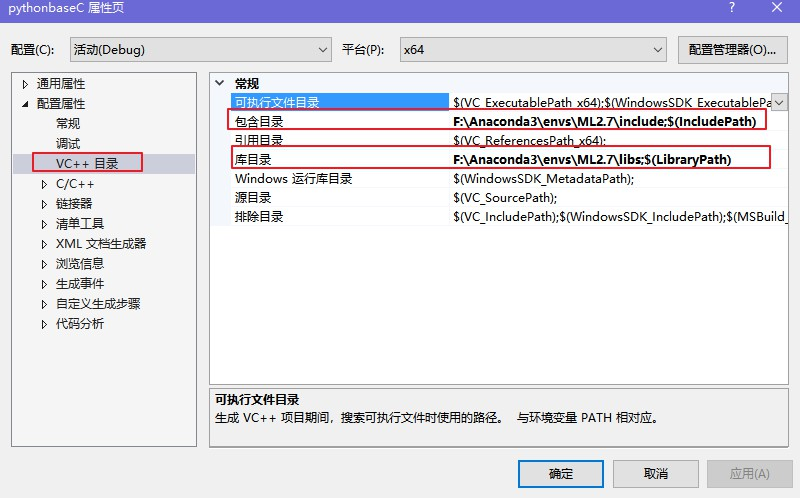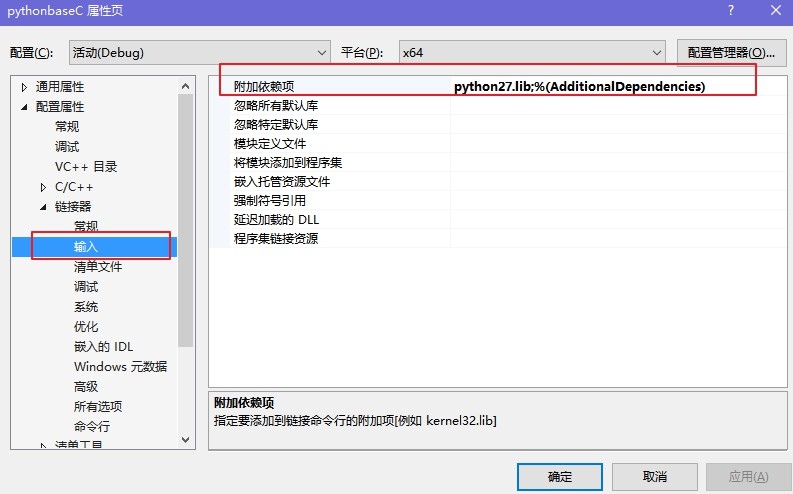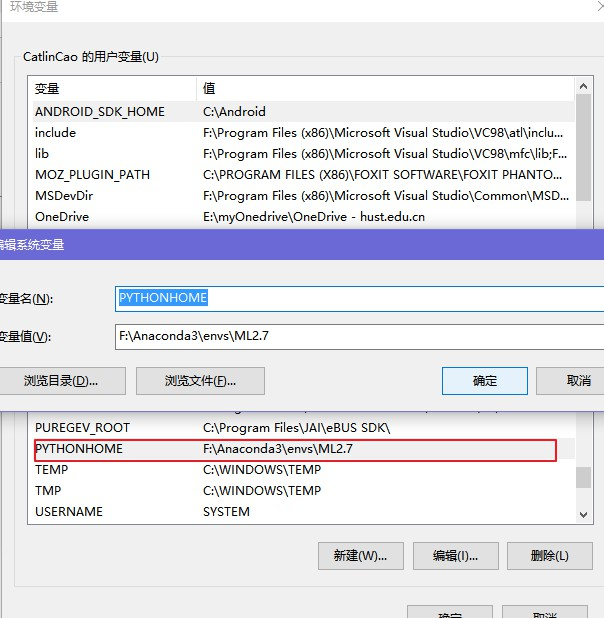在VS2013中配置python环境

1.添加头文件（.h）和库文件（.lib）的路径在“VC++目录”下的包含目录和库目录分别输入对应python的头文件和库文件路径，喵哥用的是Anaconda管理python，所以不能按照这个找路径。通常，只要在python解释器（python.exe）根目录下找到“include”和“libs”文件夹即可。

2.添加所需要的库文件——.lib和.dll3.检查系统环境变量中有无PYTHONHOME变量4.修改python里的一个头文件

#	ifdef _DEBUG

#				pragma comment(lib,"python27_d.lib")
#			else
#				pragma comment(lib,"python27.lib")
#			endif /* _DEBUG */

5.实例

python，1.py:

def add(a,b):
print ("a = " + str(a))
print ("b = " + str(b))
print ("ret = " + str(a+b))
return

C++:

#include "stdafx.h"
#include "Python.h"

int _tmain(int argc, _TCHAR* argv[])
{

// 初始化Python
//在使用Python系统前，必须使用Py_Initialize对其
//进行初始化。它会载入Python的内建模块并添加系统路
//径到模块搜索路径中。这个函数没有返回值，检查系统
//是否初始化成功需要使用Py_IsInitialized。
Py_Initialize();
// 检查初始化是否成功
if (!Py_IsInitialized())
{
return -1;
}
// 添加当前路径
//把输入的字符串作为Python代码直接运行，返回0
//表示成功，-1表示有错。大多时候错误都是因为字符串
//中有语法错误。
PyRun_SimpleString("import sys");
PyRun_SimpleString("sys.path.append('./')");
PyRun_SimpleString("import subprocess");
PyObject *pName, *pModule, *pDict, *pFunc, *pArgs;
// 载入名为1的脚本
pName = PyString_FromString("1");
pModule = PyImport_Import(pName);
if (!pModule)
{
printf("can't find 1.py");
getchar();
return -1;
}
pDict = PyModule_GetDict(pModule);
if (!pDict)
{
return -1;
}
if (!pFunc || !PyCallable_Check(pFunc))
{
getchar();
return -1;
}
// 参数进栈
pArgs = PyTuple_New(2);
//  PyObject* Py_BuildValue(char *format, ...)
//  把C++的变量转换成一个Python对象。当需要从
//  C++传递变量到Python时，就会使用这个函数。此函数
//  有点类似C的printf，但格式不同。常用的格式有
//  s 表示字符串，
//  i 表示整型变量，
//  f 表示浮点数，
//  O 表示一个Python对象。
PyTuple_SetItem(pArgs, 0, Py_BuildValue("l", 3));  //"l" (integer) [long int] :将C类型的long转换成Pyhon中的int对象。
PyTuple_SetItem(pArgs, 1, Py_BuildValue("l", 4));
// 调用Python函数
PyObject_CallObject(pFunc, pArgs);

//pFunc = PyDict_GetItemString(pDict, "redis");
//pArgs = PyTuple_New(1);
//PyTuple_SetItem(pArgs, 0, Py_BuildValue("")); //
//PyObject_CallObject(pFunc, pArgs);
Py_DECREF(pName);
Py_DECREF(pArgs);
Py_DECREF(pModule);
// 关闭Python
Py_Finalize();

return 0;
}